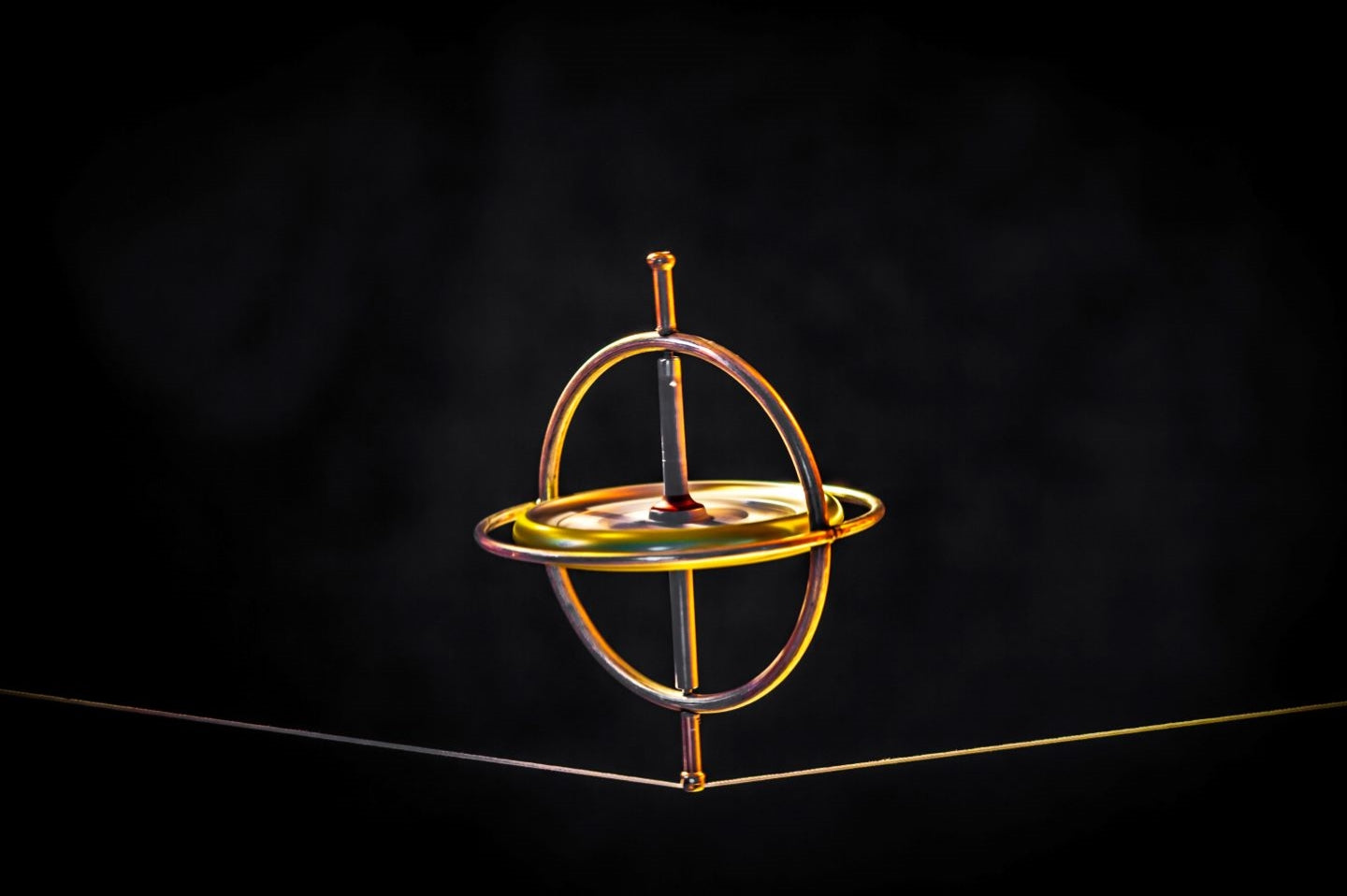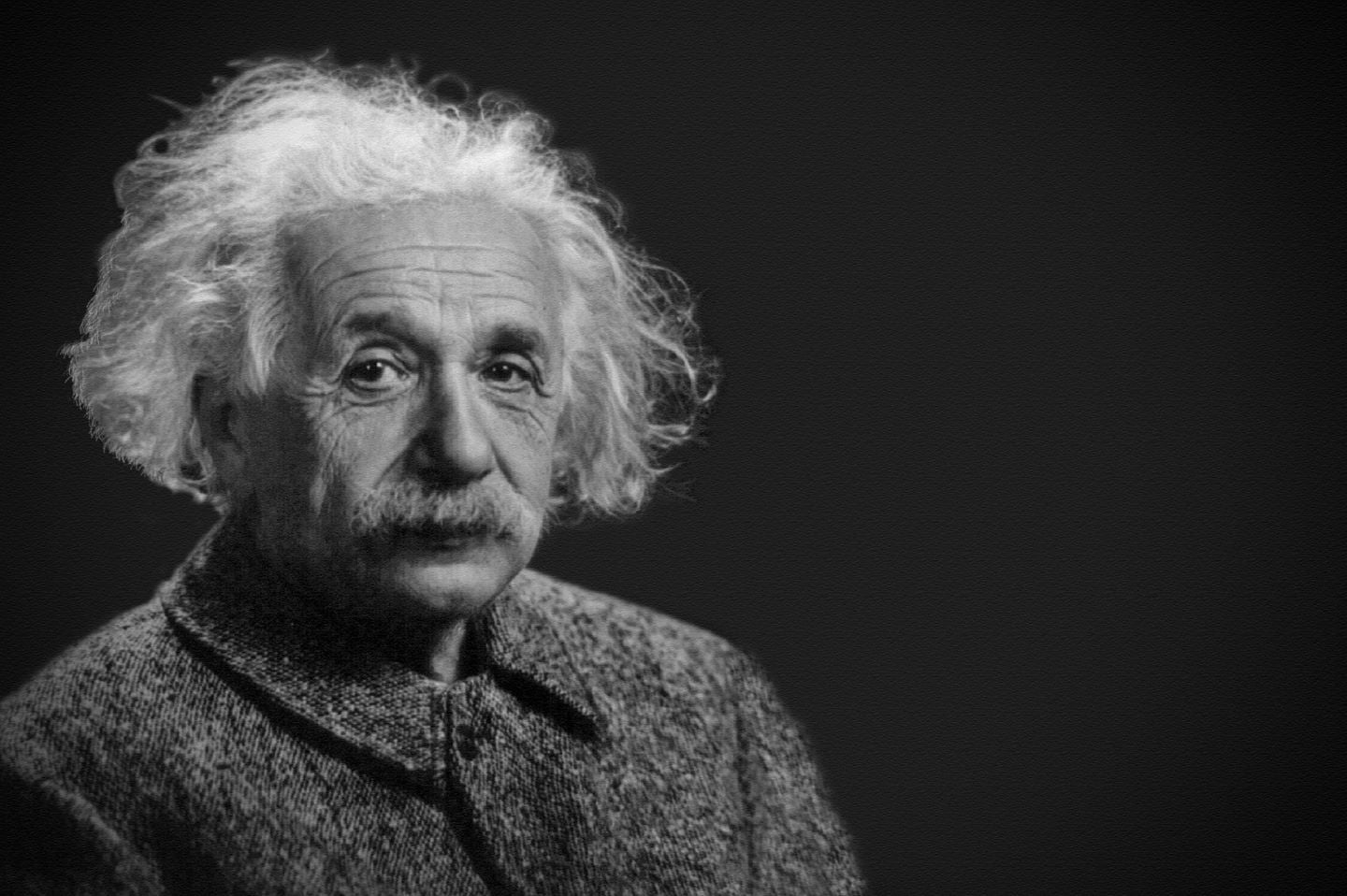# Newton's Laws of Motion## What do Newton's laws of motion say?

Also called Newtonian mechanics or classical mechanics, Newton's laws of motion are often used in the treatment of mechanics exercises. In other words, they are well known to high school students, especially those preparing for post-baccalaureate exams or the baccalaureate. Here is what you need to know about these famous Newton's laws.

## What are Newton's Laws

?

The Newton's Laws of Motion are well-known physical principles. Obviously, they were founded by Sir Isaac Newton, a British mathematician and physicist. They were first stated in 1687 in his book Philosophiae Naturalis Principia Mathematica and there are 3 of them.What exactly are they about?

In reality, these are general rules about the movement of various objects in the universe. Newton's theories thus present themselves as a clearer explanation of the nature of motion described by Aristotle. It must be said that the great British physicist used simple mathematical formulas.

Newton's Laws of Motion are not just theories. They apply in the real world. They have enabled the development of many skills. Indeed, the techniques used in spacecraft navigation are, for example, based on Newton's famous Laws of Motion. The same is true for the movements performed by billiard players.

## Newton's three laws

As mentioned earlier, Newton stated three very distinct laws. Find out which ones.

### The first law

According to the original statement of Newton's first law, "Every body perseveres in the state of rest or of uniform motion in a straight line in which it is, unless some force acts upon it, and compels it to change its state."

In a practical sense, this law states that a body or object or a body remains stationary unless it is acted upon by a force. Similarly, an object in motion tends to adopt the same speed as long as it is not acted upon by a force such as friction or gravity.

So if you throw a ball into a vacuum, it will move at the same speed unless it is confronted with the force of the wind or the force of another body like a tree. This theory of Newton's is known as the law of inertia or principle of inertia.

### The Second Law

It is called the fundamental principle of dynamics and is illustrated by the following mathematical formula: Σ F = ma. F represents the force, m the mass of the object and the acceleration of the object.

Through this formula, Newton explains the changes that a force can generate on the motion of an object. Specifically, when a force acts on a body, it causes a change in velocity, including acceleration.

It should be noted that different types of forces can act on an object. This is the case for frictional forces, gravity and electromagnetic forces. Taking this into account, this formula can be used to solve different types of problems. This is why the fundamental principle of dynamics is considered the most important physical law.

To help you understand it better, let's take the example of two round-shaped objects. One is a small rubber ball and the other is a bowling ball. In order for these two objects to roll at the same speed, the bowling ball must be pushed with a greater force because it has a greater mass. This force during the push therefore caused the speed of the bowling ball to accelerate.

If the two balls roll down a hill and land on a wall, the impact will not be the same. The bowling ball's will be harder and more damaging since it is heavier.

### The third law or principle of action-reaction

According to Isaac Newton, "Action is always equal to reaction"that is, the actions of two bodies on each other are always equal and in opposite directions." In an explicit way, we must take the example of two objects that interact. They will be named A and B. If body A exerts a force on body B, the opposite will also be true.

To understand this Newton's law better, one need only observe the recoil of a firearm. When the bullet leaves the barrel, the gun moves in the opposite direction. The same physical phenomenon occurs when you place a book on a table. The table applies a force to the book and the book applies a force to the table.

## Newton's laws in application

When should Newton's first, second, or third law be applied? In general, the principle of inertia applies when the center of gravity of a system is in uniform rectilinear motion as on the Newton's pendulum.

The second law, on the other hand, applies when the trajectory of the center of gravity of an object is not a straight line or when its velocity is not constant. It allows us to know the evolution of the motion of an object by knowing the actions of the forces that apply to it and thus to identify its direction. Finally, the third law is used to know the characteristics of the force vector of a body.